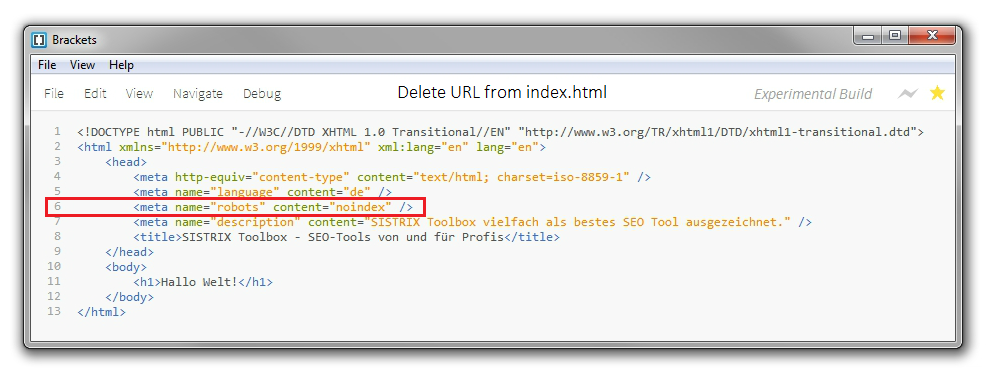## Sunday, March 25, 2007

### How to Find Over-all Database Size?

Big Portion of the database size comes from Datafiles.

To find out the size allocated to datafiles you can use below mentioned query :

1) select sum(bytes)/1024/1024 "Meg" from dba_data_files;

To get the size of all Temporary Files :

2) select nvl(sum(bytes),0)/1024/1024 "Meg" from dba_temp_files;

To get the size of Online Redo Log Files :

3) select sum(bytes)/1024/1024 "Meg" from sys.v_\$log;

So counting all of them you will get the size of the Database.

1.your command wil not give full database size....

You can use this query

How to find the size of database?
In general the size of the database is defined as total size of the physical datafiles.
Code:

select t.tablespace_name, round(a.bytes,2) tbsize,
nvl(round(c.bytes,2),'0') tbfree,
nvl(round(b.bytes,2),'0') tbused,
to_char(round(100 * (nvl(b.bytes,0)/nvl(a.bytes,1)),2)) || '%' tbusedpct,
to_char(round(100 * (nvl(c.bytes,0)/nvl(a.bytes,1)),2)) || '%' tbfreepct
from dba_tablespaces t,
(select tablespace_name, round(sum(bytes)/1024/1024,2) bytes
from dba_data_files
group by tablespace_name
union
select tablespace_name, round(sum(bytes)/1024/1024,2) bytes
from dba_temp_files
group by tablespace_name ) a,
(select e.tablespace_name, round(sum(e.bytes)/1024/1024,2) bytes
from dba_segments e
group by e.tablespace_name
union
select tablespace_name, sum(max_size) bytes
from v\$sort_segment
group by tablespace_name) b,
(select f.tablespace_name, round(sum(f.bytes)/1024/1024,2) bytes
from dba_free_space f
group by f.tablespace_name
union
select tmp.tablespace_name, (sum(bytes/1024/1024) - sum(max_size)) bytes
from dba_temp_files tmp, v\$sort_segment sort
where tmp.tablespace_name = sort.tablespace_name
group by tmp.tablespace_name) c
where
t.tablespace_name = a.tablespace_name (+)
and t.tablespace_name = b.tablespace_name (+)
and t.tablespace_name = c.tablespace_name (+)
order by t.tablespace_name

Obed Khan
OCP 9i
Mobile No +919860157699

2.This is not pretty intesting. I need to get the size of the datbase

### How can I remove a URL on my website from the Google Index?

To remove a specific URL (for example, the page http://www.example.com/page4.html) of your own website from Google’s index, there are two ...